# MultiFIT: Multiscale Fisher’s Independence Test for Multivariate Dependence

The MultiFIT package includes several functions:
1. MultiFIT: the function that runs the test of independence of two random vectors, the algorithm comprising of multiscale $$2\times2$$ univariate tests of discretized margins and multiple testing adjustments. At each resolution, recursively, tests whose p-values are below a pre-set threshold are chosen and smaller portions of the sample space that correspond to those are explored in higher resolutions. The function returns a list object that contains details of the performed tests, p-values corrected according to the selected multiple testing procedure for all tests, and global p-values for the null hypothesis that the two random vectors are independent.
2. MultiSummary: a function that returns and plots the most significant $$2\times2$$ univariate tests of discretized margins.
3. MultiTree: a function that generates a directed acyclic graph where nodes are $$2\times2$$ univariate tests of discretized margins. An edge from one test to another indicates the the latter test is performed on half the portion of the sample space on which the former was performed.

## Examples

### First Example:

#### Generate Data:

set.seed(1)
# Generate data for two random vectors, each of dimension 2, 300 observations:
n = 300
x = matrix(0, ncol = 2, nrow = n)
y = matrix(0, ncol = 2, nrow = n)

# x1 and y1 are i.i.d Normal(0,1):
x[ , 1] = rnorm(n)
y[ , 1] = rnorm(n)

# x2 is a Uniform(0,1):
x[ , 2] = runif(n)

# and y2 is depends on x2 as a noisy sine function:
y[ , 2] = sin(5 * pi * x[ , 2]) + 0.6 * rnorm(n)

plot(x[ , 1], y[ , 1], col = "grey", pch = "x", xlab = "x1", ylab = "y1")
plot(x[ , 1], y[ , 2], col = "grey", pch = "x", xlab = "x1", ylab = "y2")
plot(x[ , 2], y[ , 1], col = "grey", pch = "x", xlab = "x2", ylab = "y1")
plot(x[ , 2], y[ , 2], col = "grey", pch = "x", xlab = "x2", ylab = "y2")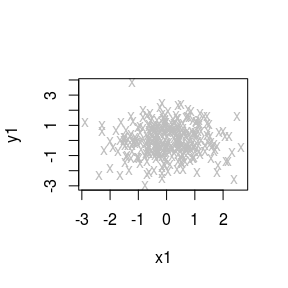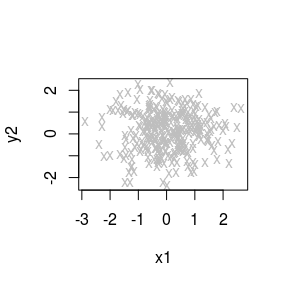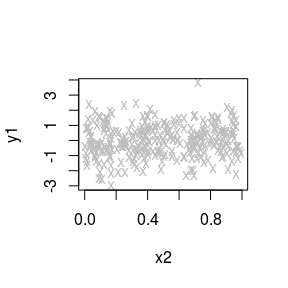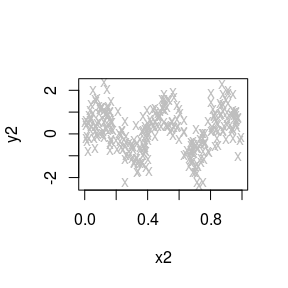#### Run the Test:

library(MultiFit)
fit = MultiFIT(x = x, y = y)
# p-values computed according to a 'holistic' strategy:
fit$p.values.holistic ## H Hcorrected ## 2.800233e-05 1.937348e-05 # p-values computed according to a 'resolution-specific' strategy: fit$p.values.resolution.specific
##           HB  HcorrectedB
## 4.923487e-05 3.406327e-05

In order to get a better sense of the workings of the function, choose verbose = TRUE:

# Data may also be transferred to the function as a single list:
xy = list(x = x, y = y)
fit = MultiFIT(xy, verbose = TRUE)
## Applying rank transformation
## Testing and computing mid-p corrected p-values:
## Resolution 0/4: performing 4 tests
## Resolution 1/4: scanning 16 cuboids, performing 32 tests
## Resolution 2/4: scanning 4 cuboids, performing 16 tests
## Resolution 3/4: scanning 16 cuboids, performing 56 tests
## Resolution 4/4: scanning 12 cuboids, performing 48 tests
## No potential parents in resolution 4 have p-values below threshold.
## Time difference of 0.02655315 secs
## Individual tests completed, computing holistic p-values...
## H: Computing all adjusted holistic p-values...
## Hcorrected: Computing all adjusted holistic p-values...
## Time difference of 0.0001893044 secs
##
## Fisher's Exact Tests:
## Global holistic p-value, Holm on p-values: 2.80023e-05
## Global resolution specific p-value, Holm on p-values: 4.92349e-05
## Global holistic p-value, Holm on p-values with mid-p correction: 1.93735e-05
## Global resolution specific p-value, Holm on p-values with mid-p correction: 3.40633e-05

The output details the number of tests performed at each resolution. The default testing method for the marginal $$2\times2$$ contingency tables is Fisher’s exact test.

The default multiple testing adjustments methods we use are Holm’s method on the original p-values (H) and Holm’s method on the mid-p corrected p-values (Hcorrected). The p-value for the global null hypothesis that $$\mathbf{x}$$ is independent of $$\mathbf{y}$$ is reported for each adjustment method, and for two strategies:

Strategy 1. A holistic approach to multiple testing. Under this strategy, one applies multiple testing control procedure on the entire set of p-values generated from all resolutions all at once, regardless of the resolution of the corresponding table.

Strategy 2. A resolution-specific approach to multiple testing. Under this strategy, one applies multiple testing control in two stages, first on the p-values within each resolution level, producing an intermediate, intra-resolution significance level for each resolution, and then in the second stage further correct these intra-resolution “p-values” over all the resolutions, which will produce a valid, corrected overall p-value for testing the global null hypothesis of independence. This strategy has the benefit that one can now “allocate” a fixed level budget to each resolution, and thus avoids the possibility of loss in power due to having many more tables tested in high resolutions than coarse ones. This method is generally more powerful than the holistic approach above for testing the global null hypothesis when a dependency structure exists in coarser resolutions.

#### Optional Stopping Rule

An additional benefit of the resolution-specific approach is that it can be implemented with early stopping so that the MultiFIT procedure can terminate as soon as there is sufficient evidence for rejecting the global null in the first few resolutions without continuing into testing on higher resolutions. This is possible because in this approach we bound the influence of tables in finer resolutions on the corrected significance level of tests in coarser resolutions. Our software implements this early stopping strategy for the resolution-specific approach to multiple testing when Holm’s method is used for intra-resolution correction along with Bonferroni’s method for cross-resolution correction. Early stopping can reduce the time complexity significantly in the presence of a global signal.

fit = MultiFIT(x = x, y = y, verbose = TRUE, apply.stopping.rule = TRUE)
## Applying rank transformation
## Testing and computing mid-p corrected p-values:
## Resolution 0/4: performing 4 tests
## Resolution 1/4: scanning 16 cuboids, performing 32 tests
## Early stopping rule met.
##
## Time difference of 0.01105762 secs
##
## Fisher's Exact Tests:
## Global resolution specific p-value, Holm on p-values: 4.92349e-05
## Global resolution specific p-value, Holm on p-values with mid-p correction: 3.40633e-05

#### An Approximate Version

Under the default strategy of setting $$p^*$$, we get that for certain alternatives, in particular those that are pervasive over the sample space and involve a large number of cuboids, the complexity of the procedure may be higher than $$O(n\log n)$$. Such large-scale, global alternatives, however, can usually be detected in coarse resolutions, and thus in practice when the algorithm is equipped with early stopping it will in fact run faster with larger $$n$$ under such alternatives.

If the practitioner wishes to ensure a strict $$O(n\log n)$$ bound on the computational complexity with or without incorporating early stopping, a simple approximate version of the multiscale Fisher’s independence test algorithm can achieve this.

Specifically, instead of including child cuboids of all cuboids with p-value less than $$p^*$$, we can include only child cuboids with p-value less than $$p^*$$ up to a maximum number of cuboids $$A$$ with the smallest Fisher’s p-values. This alternative constraint ensures that the computational cost of procedure is strictly bounded at $$O(n\log n)$$.

While under this approximation the conditions for ensuring the finite-sample guarantees are no longer satisfied, we found in practice that its statistical power.

fit = MultiFIT(x = x, y = y, verbose = TRUE, ranking.approximation = TRUE, M = 10)
## Applying rank transformation
## Testing and computing mid-p corrected p-values:
## Resolution 0/4: performing 4 tests
## Resolution 1/4: scanning 16 cuboids, performing 32 tests
## Resolution 2/4: scanning 4 cuboids, performing 16 tests
## Resolution 3/4: scanning 16 cuboids, performing 56 tests
## Resolution 4/4: scanning 12 cuboids, performing 48 tests
## Time difference of 0.02700138 secs
## Individual tests completed, computing holistic p-values...
## H: Computing all adjusted holistic p-values...
## Hcorrected: Computing all adjusted holistic p-values...
## Time difference of 0.0001871586 secs
##
## Fisher's Exact Tests:
## Global holistic p-value, Holm on p-values: 2.80023e-05
## Global resolution specific p-value, Holm on p-values: 4.92349e-05
## Global holistic p-value, Holm on p-values with mid-p correction: 1.93735e-05
## Global resolution specific p-value, Holm on p-values with mid-p correction: 3.40633e-05

#### Summarize Results (1):

In order to get a sense of the specific marginal tests that are significant at the alpha = 0.005 level, we may use the function MultiSummary:

MultiSummary(xy = xy, fit = fit, alpha = 0.05)
##
## The following tests had a p-value of less than 0.05:
## Ranked #1, Test 32: x2 and y2 | 0<=x2<0.46 (p-value=1.937348e-05)
## Ranked #2, Test 44: x2 and y2 | 0.22<=x2<0.46 (p-value=0.005378376)
## Ranked #3, Test 48: x2 and y2 | 0<=x2<0.46, -2.39<=y2<0.22 (p-value=0.01510365)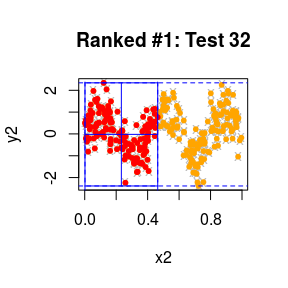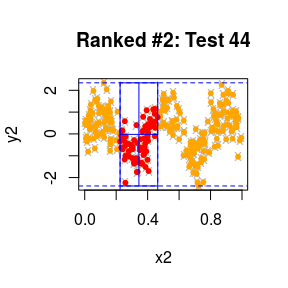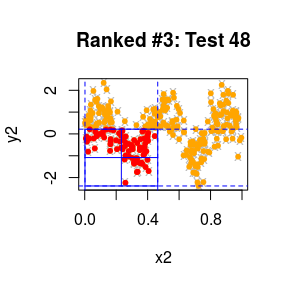In grey and orange are all data points outside the cuboid we are testing. In orange are the points that were in the cuboid if we were not to condition on the margins that are visible in the plot. In red are the points that are inside the cuboid after we condition on all the margins, including those that are visible in a plot. The blue lines delineate the quadrants along which the discretization was performed: we count the number of red points in each quadrant, treat these four numbers as a $$2\times2$$ contingency table and perform a 1-degree of freedom test of independence on it (default test: Fisher’s exact test).

#### Summarize Results (2):

We may also draw a directed acyclic graph where nodes represent tests as demonstrated above in the MultiSummary output. An edge from one test to another indicates that the latter test is performed on half the portion of the sample space on which the former was performed. Larger nodes correspond to more extreme p-values for the test depicted in it (storing the output as a pdf file):

# And plot a DAG representation of the ranked tests:
library(png)
library(qgraph)
## Registered S3 methods overwritten by 'huge':
##   method    from
##   plot.sim  BDgraph
##   print.sim BDgraph
MultiTree(xy = xy, fit = fit, filename = "first_example")
## Output stored in /tmp/RtmpzWaEpl/Rbuild41ae6760cf55/MultiFit/vignettes/first_example.pdf

We see that, in agreement with the output of the MultiSummary function, nodes 32, 44 and 48 (which correspond to tests 32, 44 and 48) are the largest compared to the other nodes.

#### Test More Cuboids:

In the default setting, p_star, the fixed threshold for $$p$$-values of tests that will be further explored in higher resolutions, is set to $$(D_x\cdot D_y\cdot \log_2(n))^{-1}$$. We may choose, e.g., p_star = 0.1, which takes longer. In this case the MultiFIT identifies more tables with adjusted p-values that are below alpha = 0.005. However, the global adjusted p-values are less extreme than when performing the MultiFIT with fewer tests:

fit1 = MultiFIT(xy, p_star = 0.1, verbose = TRUE)
## Applying rank transformation
## Testing and computing mid-p corrected p-values:
## Resolution 0/4: performing 4 tests
## Resolution 1/4: scanning 16 cuboids, performing 32 tests
## Resolution 2/4: scanning 20 cuboids, performing 76 tests
## Resolution 3/4: scanning 68 cuboids, performing 196 tests
## Resolution 4/4: scanning 88 cuboids, performing 284 tests
## No potential parents in resolution 4 have p-values below threshold.
## Time difference of 0.0518117 secs
## Individual tests completed, computing holistic p-values...
## H: Computing all adjusted holistic p-values...
## Hcorrected: Computing all adjusted holistic p-values...
## Time difference of 0.0002229214 secs
##
## Fisher's Exact Tests:
## Global holistic p-value, Holm on p-values: 9.60421e-13
## Global resolution specific p-value, Holm on p-values: 1.35673e-12
## Global holistic p-value, Holm on p-values with mid-p correction: 4.81733e-13
## Global resolution specific p-value, Holm on p-values with mid-p correction: 6.80515e-13
MultiSummary(xy = xy, fit = fit1, alpha = 0.005, plot.tests = FALSE)
##
## The following tests had a p-value of less than 0.005:
## Ranked #1, Test 100: x2 and y2 | 0.46<=x2<0.74 (p-value=4.817328e-13)
## Ranked #2, Test 32: x2 and y2 | 0<=x2<0.46 (p-value=5.705598e-05)

In order to perform the test even more exhaustively, one may:

# 1. set p_star=Inf, running through all tables up to the maximal resolution
# which by default is set to log2(n/100):
ex1 = MultiFIT(xy, p_star = 1)

# 2. set both p_star = 1 and the maximal resolution R_max = Inf.
# In this case, the algorithm will scan through higher and higher resolutions,
# until there are no more tables that satisfy the minimum requirements for
# marginal totals: min.tbl.tot, min.row.tot and min.col.tot (whose default values
# are presented below):
ex2 = MultiFIT(xy, p_star = 1, R_max = Inf,
min.tbl.tot = 25L, min.row.tot = 10L, min.col.tot = 10L)

# 3. set smaller minimal marginal totals, that will result in testing
# even more tables in higher resolutions:
ex3 = MultiFIT(xy, p_star = 1, R_max = Inf,
min.tbl.tot = 10L, min.row.tot = 4L, min.col.tot = 4L)

### A Local Signal:

MultiFIT excels in locating very localized signals.

#### Generate a Local Signal:

# Generate data for two random vectors, each of dimension 2, 800 observations:
n = 800
x = matrix(0, ncol = 2, nrow = n)
y = matrix(0, ncol = 2, nrow = n)

# x1, x2 and y1 are i.i.d Normal(0,1):
x[ , 1] = rnorm(n)
x[ , 2] = rnorm(n)
y[ , 1] = rnorm(n)

# y2 is i.i.d Normal(0,1) on most of the space:
y[ , 2] = rnorm(n)
# But is linearly dependent on x2 in a small portion of the space:
w = rnorm(n)
portion.of.space = x[ , 2] > 0 & x[ , 2] < 0.7 & y[ , 2] > 0 & y[ , 2] < 0.7
y[portion.of.space, 2] = x[portion.of.space, 2] + (1 / 12) * w[portion.of.space]
xy.local = list(x = x, y = y)

#### Search for It and Summarize the Results:

Truly local signals may not be visible to our algorithm in resolutions that are lower than the one that the signal is embedded in. In order to cover all possible tests up to a given resolution (here: resolution 4), we use the parameter R_star = 4 (from resolution 5 onward, only tables with p-values below p_star will be further tested):

fit.local = MultiFIT(xy = xy.local, R_star = 4, verbose = TRUE)
## Applying rank transformation
## Testing and computing mid-p corrected p-values:
## Resolution 0/6: performing 4 tests
## Resolution 1/6: scanning 16 cuboids, performing 32 tests
## Resolution 2/6: scanning 128 cuboids, performing 160 tests
## Resolution 3/6: scanning 640 cuboids, performing 640 tests
## Resolution 4/6: scanning 2560 cuboids, performing 2240 tests
## Resolution 5/6: scanning 140 cuboids, performing 524 tests
## Resolution 6/6: scanning 16 cuboids, performing 64 tests
## No pairs of margins in resolution 6 had enough observations to be tested.
## Time difference of 0.1756155 secs
## Individual tests completed, computing holistic p-values...
## H: Computing all adjusted holistic p-values...
## Hcorrected: Computing all adjusted holistic p-values...
## Time difference of 0.0007996559 secs
##
## Fisher's Exact Tests:
## Global holistic p-value, Holm on p-values: 1.61645e-05
## Global resolution specific p-value, Holm on p-values: 7.9529e-05
## Global holistic p-value, Holm on p-values with mid-p correction: 8.11708e-06
## Global resolution specific p-value, Holm on p-values with mid-p correction: 3.99359e-05
MultiSummary(xy = xy.local, fit = fit.local, plot.margin = TRUE, pch = "")
##
## The following tests had a p-value of less than 0.05:
## Ranked #1, Test 2928: x2 and y2 | -0.06<=x2<0.7, -0.06<=y2<0.62 (p-value=8.117077e-06)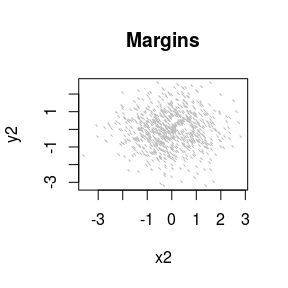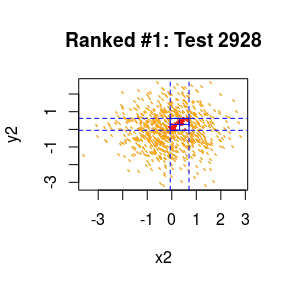### A Signal that is Spread Between More than 2 Margins:

MultiFit also has the potential to identify complex conditional dependencies in multivariate signals.

#### Generate Data and Examine Margins:

Take $$\mathbf{x}$$ and $$\mathbf{y}$$ to be each of three dimensions, with 800 data points. We first generate a marginal circle dependency: $$x_1$$, $$y_1$$, $$x_2$$, and $$y_2$$ are all i.i.d standard normals. Take $$x_3=\cos(\theta)+\epsilon$$, $$y_3=\sin(\theta)+\epsilon'$$ where $$\epsilon$$ and $$\epsilon'$$ are i.i.d $$\mathrm{N}(0,(1/10)^2)$$ and $$\theta\sim \mathrm{Uniform}(-\pi,\pi)$$. I.e., the original dependency is between $$x_3$$ and $$y_3$$.

# Marginal signal:
n = 800
x = matrix(0, ncol = 3, nrow = n)
y = matrix(0, ncol = 3, nrow = n)

# x1, x2, y1 and y2 are all i.i.d Normal(0,1)
x[ , 1] = rnorm(n)
x[ , 2] = rnorm(n)
y[ , 1] = rnorm(n)
y[ , 2] = rnorm(n)

# x3 and y3 form a noisy circle:
theta = runif(n, -pi, pi)
x[ , 3] = cos(theta) + 0.1 * rnorm(n)
y[ , 3] = sin(theta) + 0.1 * rnorm(n)

par(mfrow = c(3, 3))
par(mgp = c(0, 0, 0))
par(mar = c(1.5, 1.5, 0, 0))
for (i in 1:3) {
for (j in 1:3) {
plot(x[ , i], y[ , j], col = "black", pch = 20, xlab = paste0("x", i), ylab = paste0("y", j),
xaxt = "n", yaxt = "n")
}
}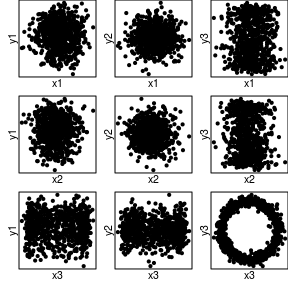Next, rotate the circle in $$\pi/4$$ degrees in the $$x_2$$-$$x_3$$-$$y_3$$ space by applying:

$$\left[\begin{matrix}\cos(\pi/4) & -sin(\pi/4) & 0\\\sin(\pi/4) & cos(\pi/4) & 0\\ 0 & 0 & 1\end{matrix}\right]\left[\begin{matrix}| & | & |\\X_2 & X_3 & Y_3\\| & | & |\end{matrix}\right]$$

I.e., once rotated the signal is ‘spread’ between $$x_2$$, $$x_3$$ and $$y_3$$, and harder to see through the marginal plots.

# And now rotate the circle:
phi = pi/4
rot.mat =
matrix(
c(cos(phi), -sin(phi),  0,
sin(phi),  cos(phi),  0,
0,         0,         1),
nrow = 3,
ncol = 3
)
xxy = t(rot.mat %*% t(cbind(x[ , 2], x[ , 3], y[ , 3])))

x.rtt = matrix(0, ncol = 3, nrow = n)
y.rtt = matrix(0, ncol = 3, nrow = n)

x.rtt[ , 1] = x[ , 1]
x.rtt[ , 2] = xxy[ , 1]
x.rtt[ , 3] = xxy[ , 2]
y.rtt[ , 1] = y[ , 1]
y.rtt[ , 2] = y[ , 2]
y.rtt[ , 3] = xxy[ , 3]

par(mfrow=c(3, 3))
par(mgp=c(0, 0, 0))
par(mar=c(1.5, 1.5, 0, 0))
for (i in 1:3) {
for (j in 1:3) {
plot(x.rtt[ , i], y.rtt[ , j], col = "black", pch = 20, xlab = paste0("x", i),
ylab = paste0("y", j), xaxt = "n", yaxt = "n")
}
}

xy.rtt.circ = list(x = x.rtt, y = y.rtt)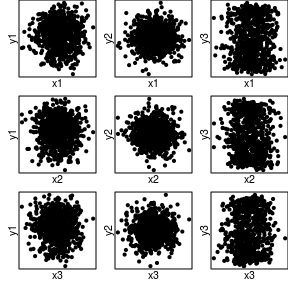#### Run the Test and Summarize the Data:

fit.rtt.circ = MultiFIT(xy = xy.rtt.circ, R_star = 2, verbose = TRUE)
## Applying rank transformation
## Testing and computing mid-p corrected p-values:
## Resolution 0/6: performing 9 tests
## Resolution 1/6: scanning 36 cuboids, performing 108 tests
## Resolution 2/6: scanning 432 cuboids, performing 756 tests
## Resolution 3/6: scanning 76 cuboids, performing 549 tests
## Resolution 4/6: scanning 56 cuboids, performing 450 tests
## Resolution 5/6: scanning 48 cuboids, performing 387 tests
## Resolution 6/6: scanning 8 cuboids, performing 72 tests
## No pairs of margins in resolution 6 had enough observations to be tested.
## Time difference of 0.1145997 secs
## Individual tests completed, computing holistic p-values...
## H: Computing all adjusted holistic p-values...
## Hcorrected: Computing all adjusted holistic p-values...
## Time difference of 0.0004496574 secs
##
## Fisher's Exact Tests:
## Global holistic p-value, Holm on p-values: 2.76779e-07
## Global resolution specific p-value, Holm on p-values: 7.85792e-07
## Global holistic p-value, Holm on p-values with mid-p correction: 1.98468e-07
## Global resolution specific p-value, Holm on p-values with mid-p correction: 5.63462e-07
MultiSummary(xy = xy.rtt.circ, fit = fit.rtt.circ, alpha = 0.00005)
##
## The following tests had a p-value of less than 5e-05:
## Ranked #1, Test 684: x3 and y3 | -2.7<=x2<-0.07, -1.23<=y3<-0.11 (p-value=1.98468e-07)
## Ranked #2, Test 1746: x3 and y3 | -0.65<=x2<-0.07, -2.91<=x3<0.01, -0.11<=y3<1.23 (p-value=8.789752e-07)
## Ranked #3, Test 1206: x3 and y3 | 0.6<=x2<2.76, -1.23<=y3<-0.11 (p-value=3.700669e-05)
## Ranked #4, Test 708: x2 and y3 | 0.01<=x3<3.01, -1.23<=y3<-0.11 (p-value=4.916867e-05)`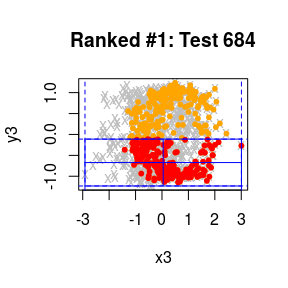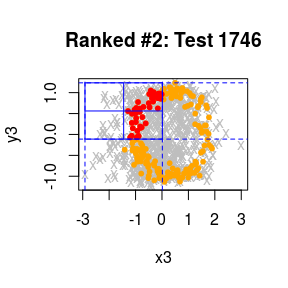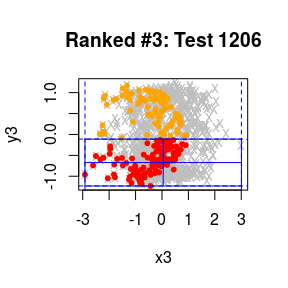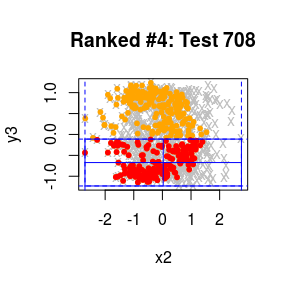Notice how the signal is detected both in the $$x_3$$-$$y_3$$ plane and the $$x_2$$-$$y_3$$ plane.